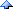# 77家的会客厅2010

==和equals对擂
Weather:天晴了，不下雨了

1. 检查对象是否相等

//: Equivalence.java

public class Equivalence {
public static void main(String[] args) {
Integer n1 = new Integer(47);
Integer n2 = new Integer(47);
System.out.println(n1 == n2);
System.out.println(n1 != n2);
}
} ///:~

//: EqualsMethod.java

public class EqualsMethod {
public static void main(String[] args) {
Integer n1 = new Integer(47);
Integer n2 = new Integer(47);
System.out.println(n1.equals(n2));
}
} ///:~

//: EqualsMethod2.java

class Value {
int i;
}

public class EqualsMethod2 {
public static void main(String[] args) {
Value v1 = new Value();
Value v2 = new Value();
v1.i = v2.i = 100;
System.out.println(v1.equals(v2));
}
} ///:~

public class TestString {
public static void main(String[] args) {
String s1 = "Monday";
String s2 = "Monday";
}
}

1. 来自 String 的忧虑

String 是 final 类，它的值不可变。

public class TestString {
public static void main(String[] args) {
String s1 = "Monday";
String s2 = "Monday";
if (s1 == s2)
System.out.println("s1 == s2");
else
System.out.println("s1 != s2");
}
}

== 分明是在说：s1 与 s2 引用同一个 String 对象 -- "Monday"!

2. 千变万化的 String

public class TestString {
public static void main(String[] args) {
String s1 = "Monday";
String s2 = new String("Monday");
if (s1 == s2)
System.out.println("s1 == s2");
else
System.out.println("s1 != s2");
if (s1.equals(s2))
System.out.println("s1 equals s2");
else
System.out.println("s1 not equals s2");
}
}

s1 != s2
s1 equals s2

s1 s2分别引用了两个"Monday"String对象

3. 在 String 的游泳池中游泳

“我要一个新的！不要旧的！”与是一个新的"Monday"Sting对象被创

4. 继续潜水

public class TestString {
public static void main(String[] args) {
String s1 = "Monday";
String s2 = new String("Monday");
s2 = s2.intern();
if (s1 == s2)
System.out.println("s1 == s2");
else
System.out.println("s1 != s2");
if (s1.equals(s2))
System.out.println("s1 equals s2");
else
System.out.println("s1 not equals s2");
}
}

s1 == s2
s1 equals s2

5. == 与 equals() 的争斗
String 是个对象，要对比两个不同的String对象的值是否相同

String s2 = new String("Monday").intern();(04/19) 包二奶和“绿帽子”的成本